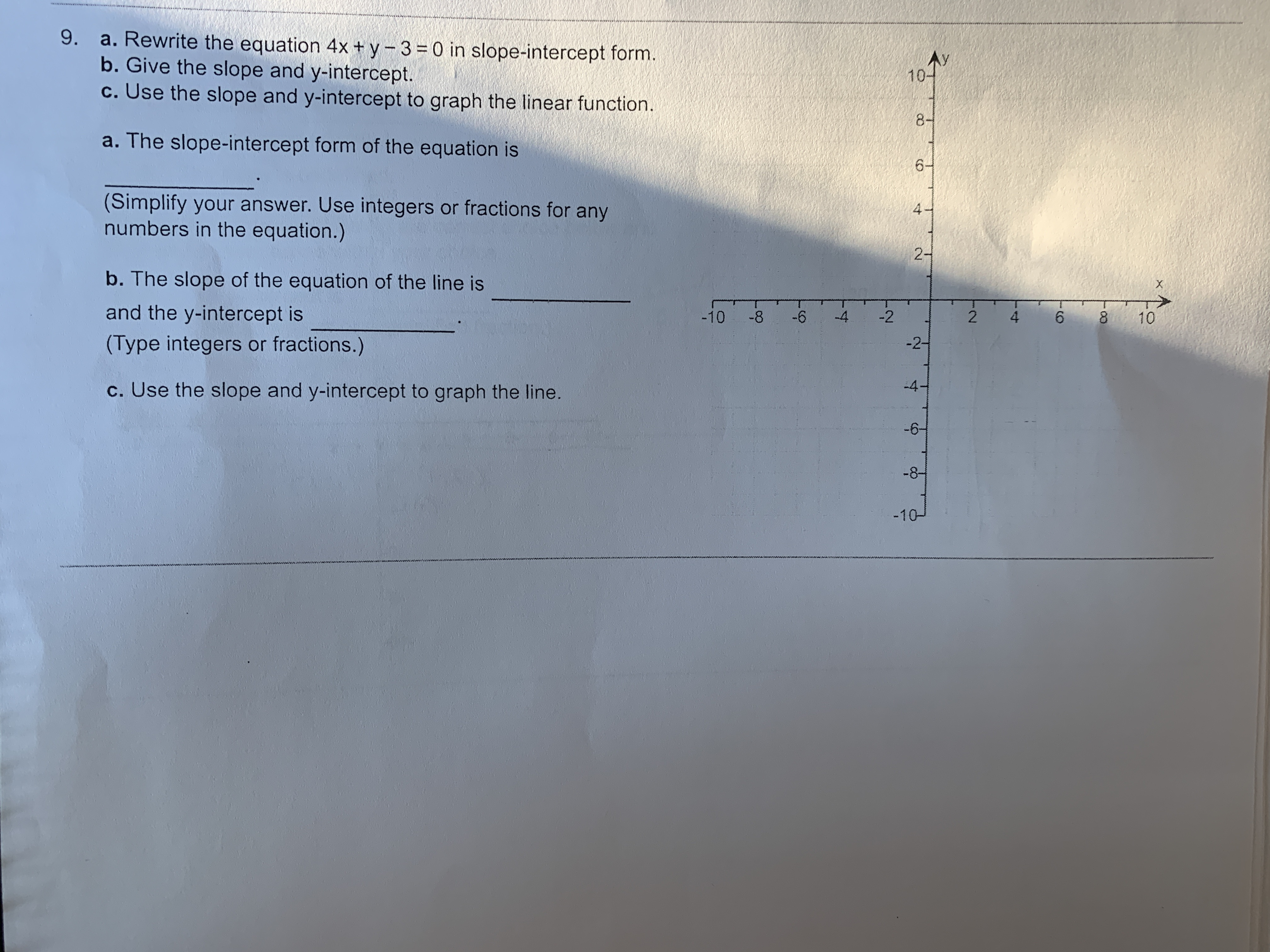# 0o49. a. Rewrite the equation 4x + y 3 0 in slope-intercept form.b. Give the slope and y-intercept.C.Use the slope and y-intercept to graph the linear function.108-a. The slope-intercept form of the equation is6(Simplify your answer. Use integers or fractions for anynumbers in the equation.)42-b. The slope of the equation of the line isXand the y-intercept is-10-8-64-22610-2-(Type integers or fractions.)-4-c. Use the slope and y-intercept to graph the line.-6--8--10

Question

Can someone help me with this problem?help_outlineImage Transcriptionclose0o 4 9. a. Rewrite the equation 4x + y 3 0 in slope-intercept form. b. Give the slope and y-intercept. C.Use the slope and y-intercept to graph the linear function. 10 8- a. The slope-intercept form of the equation is 6 (Simplify your answer. Use integers or fractions for any numbers in the equation.) 4 2- b. The slope of the equation of the line is X and the y-intercept is -10 -8 -6 4 -2 2 6 10 -2- (Type integers or fractions.) -4- c. Use the slope and y-intercept to graph the line. -6- -8- -10 fullscreen
check_circleExpert Solution
Step 1

Consider the given equation.

Step 2

Part (a)

Convert the above equation in the slope intercept from, we get

Step 3

Part (b)

From the part (a),

Slope = -...

### Want to see the full answer?

See Solution

#### Want to see this answer and more?

Solutions are written by subject experts who are available 24/7. Questions are typically answered within 1 hour*

See Solution
*Response times may vary by subject and question
Tagged in

### Other